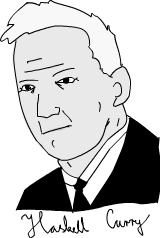# Currying

## Why need Currying?

Do you remember Haskell Curry?What a coincidence, there is a person named Haskell Curry. So that the programming language `Haskell` applies Currying automatically. But, is that wired, why do we need currying, and why is it so important that `Haskell` applies currying on all functions by default. Is currying just a returning a partial configured function?

Let's look at some codes in Haskell:

``````max 3 4
(max 3) 4
``````

Both of them return `4`, but what is the purposes of the second one?

Try to use the conception of high-order function to explain it. What? It's hard to understand `Haskell`, let's look at it in JavaScript.

### Solving a problem

#### 1. Write a function that concats each string in array, like `f(['1','2']) => '12'`

All right, if we don't use currying, how could we implement this function? Yes, using `reduce`:

``````var concatArray = function(chars){
return chars.reduce(function(a, b){
return a.concat(b);
});
}
concat(['1','2','3']) // => '123'
``````

Easy, right?

#### 2. Write a function that concats each string in array, before concating, increases each number by 1

``````var concatArray = function(chars, inc){
return chars.map(function(char){
return (+char)+inc + '';
}).reduce(function(a,b){
return a.concat(b)
});
}
console.log(concatArray(['1','2','3'], 1))// => '234'
``````

#### 3. Refactor this function, before concating, multiply each number by 2

``````var multiple = function(a, b){
return +a*b + ''
}
var concatArray = function(chars, inc){
return chars.map(function(char){
return multiple(char, inc);
}).reduce(function(a,b){
return a.concat(b)
});
}
console.log(concatArray(['1','2','3'], 2)) // => '246'
``````

Have you noticed the problem? If we want to subtract each number by 2, we have to replace the anonymous `multiple` function in `map`'s parameter. Currently, we may not use `concatArray` with addition, multiplying or substrction at same time. How can we extract them from `concatArray`? Let's compare the implementation using Currying.

### Curring the interface

``````var multiple = function(a){
return function(b){
return +b*a + ''
}
}

var plus = function(a){
return function(b){
return (+b)+a + ''
}
}
var concatArray = function(chars, stylishChar){
return chars.map(stylishChar)
.reduce(function(a,b){
return a.concat(b)
});
}
console.log(concatArray(['1','2','3'], multiple(2)))
console.log(concatArray(['1','2','3'], plus(2)))
``````

The different is:

1. we extract the functions that modify each character in array, treat them as the parameter, pass them into `concatArray`
2. The currying functions can be configured and passed into `concatArray`. The benefits of making interface fluently is obvious. No matter we invoke them at outer level:

``````concatArray(['1','2','3'], multiple(2))
``````

Or we pass them into `map`:

``````chars.map(stylishChar)
``````

These interfaces become more clear, right?

This is a conception of functional programming, that is we can combinate a new function with the functions we already have. Each time when the curring consumes a function, it will return a new function that is partial configured. This combination brings us a flexible approach to make interface fluently.

### Automatic Currying

In `Haskell`, a function will become currying automaticly:

``````max 3 4
``````

the code above is equivalent to:

`````` (max 3) 4
``````

Look at the type of `max` and `max 3`:

``````ghci> :t max
max :: Ord a => a -> a -> a
``````

Understand? `Ord a =>` means the parameter `a` can be ordered, so that the type of `max` is: given `a`, it returns `a -> a`. While, look at the type of `max 3`:

``````ghci> :t max 3
(Num a, Ord a) => a -> a
``````

`(Num a, Ord a) =>` means the parameter `a` can be a `Num` or can be ordered. Because it has a parameter, so `max 3` is also a function: given a `Ord` or `Num`, this function returns another `Ord` or `Num`.

Is this more clear? In Haskell, passing a parameter, if this function has more parameters, it will return a new function that can handle the remain parameters. This is called automatic currying.

However, Currying is not automatic in JavaScript (and most other languages). If we give a function only part of its parameter, the function treats other parameters as `undefined`, and it will not return a new function handling remain parameters.

``````function willNotCurry(a, b, c) {
console.log(a, b, c)
return a*b-c;
}
willNotCurry(1)
// => NaN
// => 1 undefined undefined
``````

We can use the automatic currying library ramda to make our example perfect:

``````var multiple = ramda.curry(function(a, b){
return +b*a + ''
})
var plus = ramda.curry(function(a, b){
return (+b)+a + ''
})
``````

code